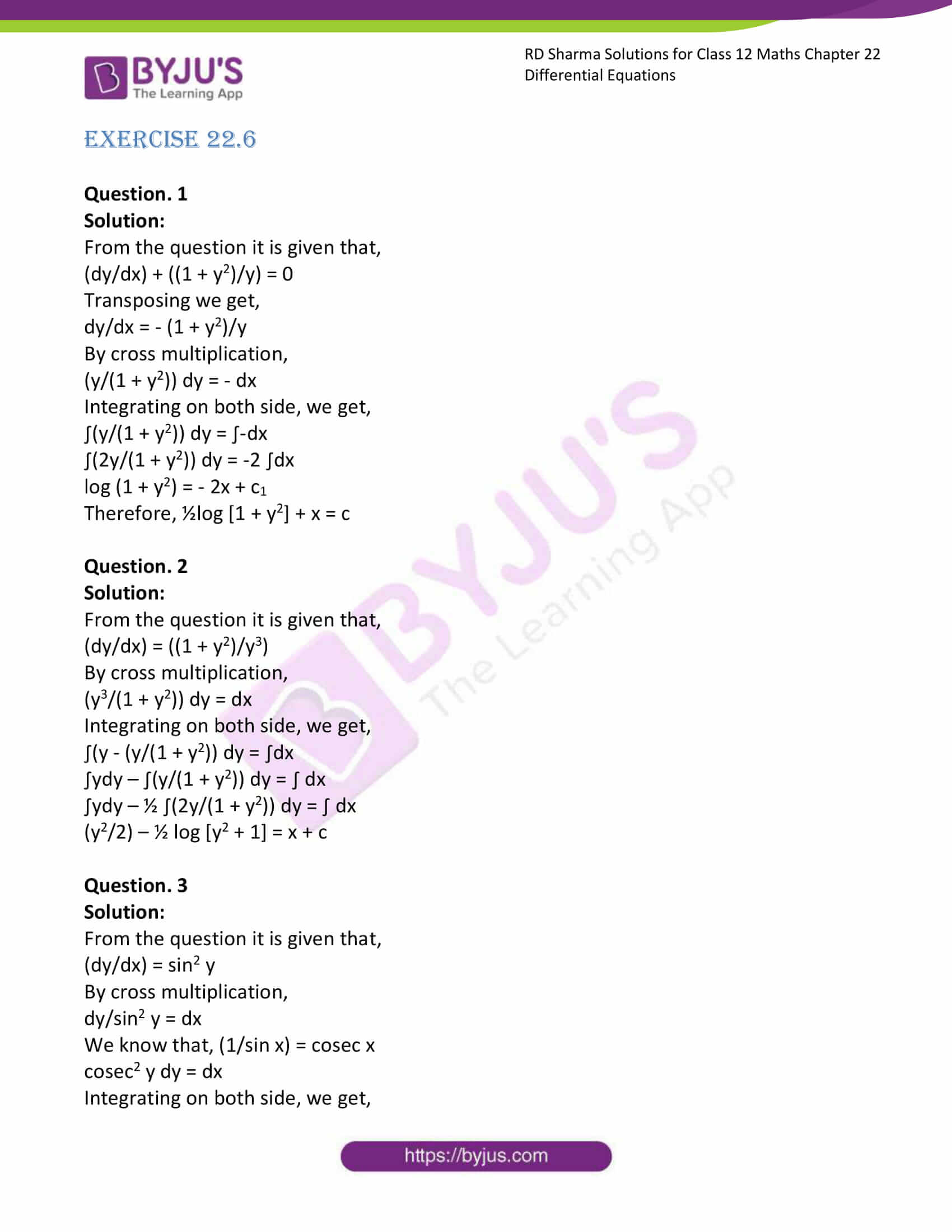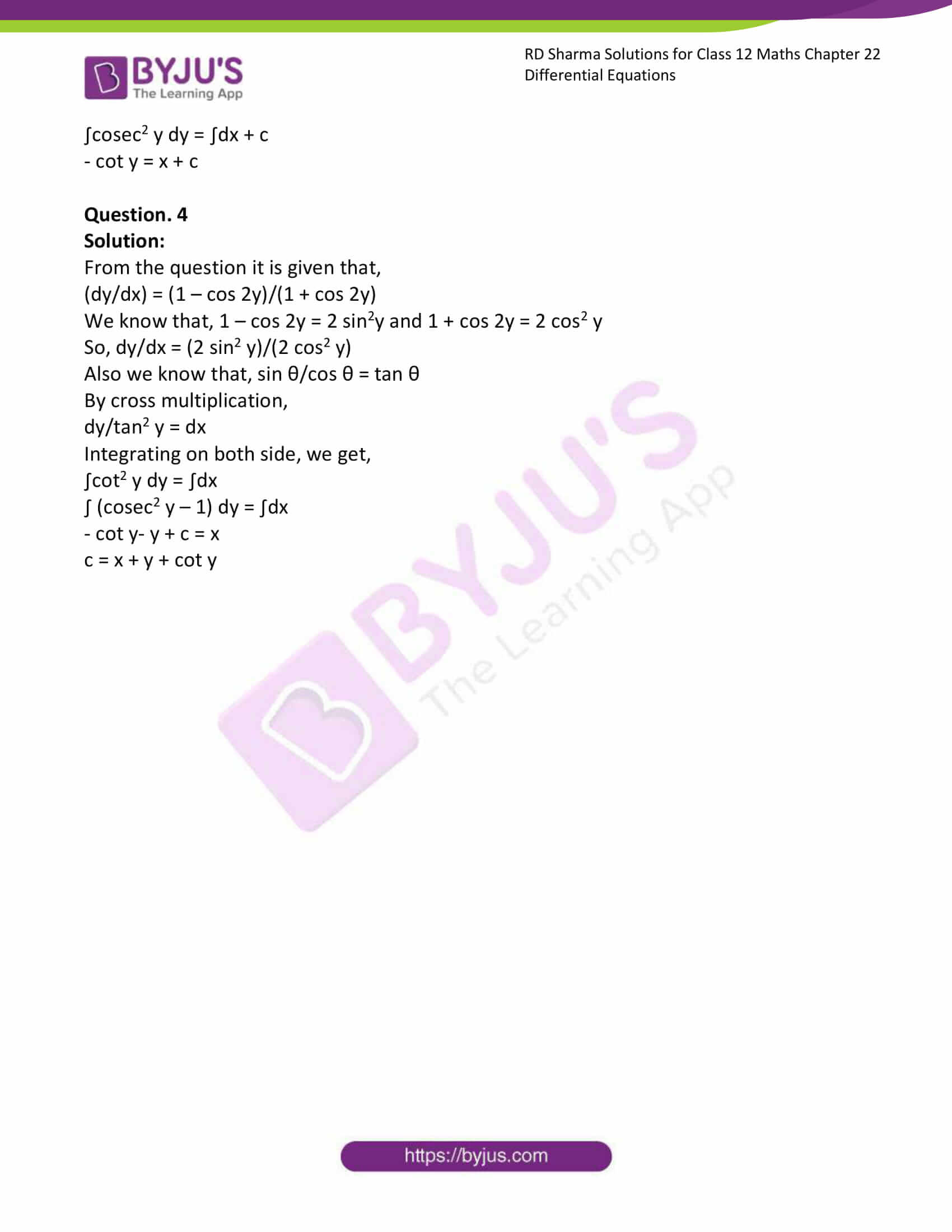# RD Sharma Solutions Class 12 Differential Equations Exercise 22.6

RD Sharma Solutions for Class 12 Maths Exercise 22.6 Chapter 22 Differential Equations are available here. If students want to score higher in Maths, it requires the right amount of practice for every topic. More marks can be achieved by referring to RD Sharma Solutions for Class 12 Maths Chapter 22. Problems are solved step by step by BYJU’S experts with neat explanations.

## Download PDF of RD Sharma Solutions for Class 12 Maths Chapter 22 Exercise 6### Access RD Sharma Solutions for Class 12 Maths Chapter 22 Exercise 6

EXERCISE 22.6

Question. 1

Solution:

From the question it is given that,

(dy/dx) + ((1 + y2)/y) = 0

Transposing we get,

dy/dx = – (1 + y2)/y

By cross multiplication,

(y/(1 + y2)) dy = – dx

Integrating on both side, we get,

∫(y/(1 + y2)) dy = ∫-dx

∫(2y/(1 + y2)) dy = -2 ∫dx

log (1 + y2) = – 2x + c1

Therefore, ½log [1 + y2] + x = c

Question. 2

Solution:

From the question it is given that,

(dy/dx) = ((1 + y2)/y3)

By cross multiplication,

(y3/(1 + y2)) dy = dx

Integrating on both side, we get,

∫(y – (y/(1 + y2)) dy = ∫dx

∫ydy – ∫(y/(1 + y2)) dy = ∫ dx

∫ydy – ½ ∫(2y/(1 + y2)) dy = ∫ dx

(y2/2) – ½ log [y2 + 1] = x + c

Question. 3

Solution:

From the question it is given that,

(dy/dx) = sin2 y

By cross multiplication,

dy/sin2 y = dx

We know that, (1/sin x) = cosec x

cosec2 y dy = dx

Integrating on both side, we get,

∫cosec2 y dy = ∫dx + c

– cot y = x + c

Question. 4

Solution:

From the question it is given that,

(dy/dx) = (1 – cos 2y)/(1 + cos 2y)

We know that, 1 – cos 2y = 2 sin2y and 1 + cos 2y = 2 cos2 y

So, dy/dx = (2 sin2 y)/(2 cos2 y)

Also we know that, sin θ/cos θ = tan θ

By cross multiplication,

dy/tan2 y = dx

Integrating on both side, we get,

∫cot2 y dy = ∫dx

∫ (cosec2 y – 1) dy = ∫dx

– cot y- y + c = x

c = x + y + cot y